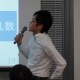3.8k

# 論文読んだ「Winner’s Curse: Bias Estimation for Total Effects of Features in Online Controlled Experiments 」

Winner’s Curse: Bias Estimation for Total Effects of Features in Online Controlled ExperimentsMinyong Lee (Airbnb); Milan Shen (Airbnb) (KDD 2018)## Shinichi Takayanagi

September 26, 2018

## Transcript

1. ### Winner’s Curse: Bias Estimation for Total Effects of Features in

Online Controlled Experiments Minyong Lee (Airbnb); Milan Shen (Airbnb) (KDD 2018)   @_stakaya 
2. ###  • !2AB)Music.1 -#43+( • "%'0AB)Music .15#43+( • &* 

!2”\$,43/”    2
3. ### • !2AB)Music.1 -#43+( • "%'0AB)Music .15#43+( • &*  !2”\$,43/”

    3
4. ###  • >,AB*)%5;4Bias <6%5?7 \$  – Winner‘s Curse Bias[email protected]

• 8+&(2 –#9'/0*:- .4  3Business Impact1  • AirbnbAB" ! 4
5. ###   •  3<AB\$"&     )%*

–@A/B\$"&; #' → Gaussian 28 79B0.1 ;6!; / 2,NG –CA4  >5?: +- Wiki() =… 5
6. ### • n7"!#0  • i8("!#;*Metrics/& –5 /&),90  –… 62OK

• <>631- –5'% 2 • +4&= : –31\$ .2 +4&=    6
7. ###   • #& – b,# %( .+ – -*"

!(  • ATotal True Effect – *" – A\$)' \$)' 7
8. ###   • AExpected Total True Effect • ATotal Estimated

Effect – Total   –     8

10. ### upward bias   10   i∈A  

A      
11. ### upward bias   11 X_i > b_i ¥sigma_i I()

    
12. ### upward bias   12 I(A) = 1– I(Not(A)) \$

 & X_i ≦ b_i ¥sigma_i'#I() !   %"



16. ### upaward bias   • # Bias • " •

  –*\$/(0%- &)  –!(  +'  •  ., i=1, …,n  16
17. ### Selection bias with fixed p-values • p  Bias 

•    Bias 17
18. ###  • Bias  –     –

    18

20. ### "%  • Zhong and Prentice , Efron , and

Xu, Craiu and Sun A Bias  •   20    Gaussian '#&  !\$  
21. ###  • Zhong and Prentice , Efron , and Xu,

Craiu and Sun A Bias 21
22. ### Bootstrap • Total true effect"   !  •

  # 22
23. ###  • n=30 • • σ24\$&5(shape=3, scale=1) .+ • ,3*

0  • … /6'(2%AB1,000) ! #"\$-1 23

26. ### Code 26 library("ggplot2") theme_set(theme_grey(base_size=28)) #   # Zi|(−1.5 <

Zi < 2) where Zi ∼ N(0.2,0.7^2) a <- qnorm(runif(10^5, pnorm(-1.5, mean=0.2, sd=0.7), pnorm(2, mean=0.2, sd=0.7)), mean=0.2, sd=0.7) ggplot(data.frame(value=z), aes(x = value, y = ..density..)) + geom_density(aes(alpha = 0.2), color="#4CAF50", fill="#4CAF50", show.legend=FALSE) + xlim(c(-2.5, 2.5)) + theme_grey(base_size=28) #    Code   # σ^2 rform the inverse gamma distribution with shape parameter3 and scale param 1 sigma <- sqrt(1/rgamma(10^5, shape=3, scale=1)) ggplot(data.frame(value=sigma), aes(x = value, y = ..density..)) + geom_density(aes(alpha = 0.2), color="#4CAF50", fill="#4CAF50", show.legend=FALSE) + xlim(c(0, 2)) + theme_grey(base_size=28)

30. ### Code 30 set.seed(71) size <- 30 a <- qnorm(runif(size, pnorm(-1.5,

mean=0.2, sd=0.7), pnorm(2, mean=0.2, sd=0.7)), mean=0.2, sd=0.7) sigma <- sqrt(1/rgamma(size, shape=3, scale=1)) b <- qnorm(0.95, mean=0, sd=1) effect <- list() for(i in seq_len(10^3)){ x <- purrr::map_dbl(seq_len(size), ~ rnorm(1, mean=a[.x], sd=sigma[.x])) binary_win <- as.numeric(x/sigma > b) effect[[length(effect) + 1]] <- data.frame( # S_{A} sa=sum(x*binary_win), # T_{A} ta=sum(x*binary_win) - sum(sigma * dnorm((sigma * b - x)/sigma)), # T_{A, cond} tc=sum(x*binary_win) - sum(sigma * dnorm((sigma * b - x)/sigma)/(1 - pnorm((sigma * b - x)/sigma))*binary_win), # True effect te=sum(a*binary_win) ) } df <- dplyr::bind_rows(effect) # The total estimated effect v.s. The total true effect ggplot(df, aes(x=te, y=sa)) + geom_point() + geom_abline(slope=1, intercept=0) # The expected total true effect (conditional) v.s. The total true effect ggplot(df, aes(x=te, y=tc)) + geom_point() + geom_abline(slope=1, intercept=0) # The expected total true effect v.s. The total true effect ggplot(df, aes(x=te, y=ta)) + geom_point() + geom_abline(slope=1, intercept=0)
31. ###   • Market Dynamics team  31 Figure 6

Holdout  
32. ### Experimentation Reporting Framework (ERF) At Airbnb • 100  Product

Team   • 3,000 Metrics Monitoring • Winner‘s Curse Bias  \$!0 32 Figure 8 # , "
33. ### Aibnb • 53=LDαθG0 >  –'%( 7?5,\$!*Dθ –8S7?=LDα • 9JRF2

 '%(B /;  –MetricsNeutral *&" .)COA/B'% ([email protected]P'%(B/1 • Holdout#-+E< –MQ:[email protected]6AKI< 33
34. ###  •  Bradley Efron. 2011. TweedieâĂŹs formula and selection

bias. J. Amer. Statist. Assoc. 106, 496 (Dec. 2011), 1602–1614. •  Will Moss. 2014. Experiment reporting framework. (May 2014). Retrieved February 16, 2017 from http://nerds.airbnb.com/experiment-reporting- framework •  Jan Overgoor. 2014. Experiments at Airbnb. (May 2014). Retrieved February 16, 2017 from http://nerds.airbnb.com/experiments-at-airbnb •  Lizhen Xu, Radu V Craiu, and Lei Sun. 2011. Bayesian methods to overcome the winner’s curse in genetic studies. The Annals of Applied Statistics (2011) •  Hua Zhong and Ross L Prentice. 2008. Bias-reduced estimators and confidence intervals for odds ratios in genome-wide association studies. Biostatistics 9, 4 (Oct. 2008), 621–634. 34